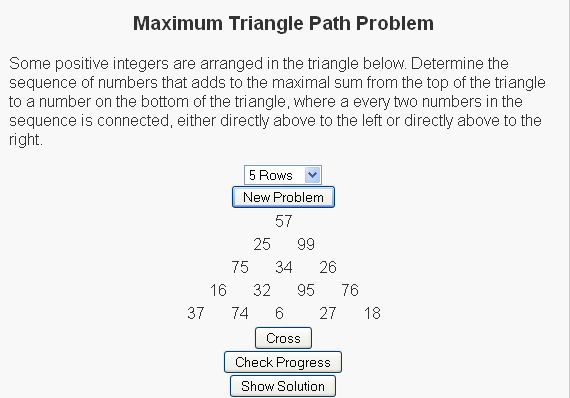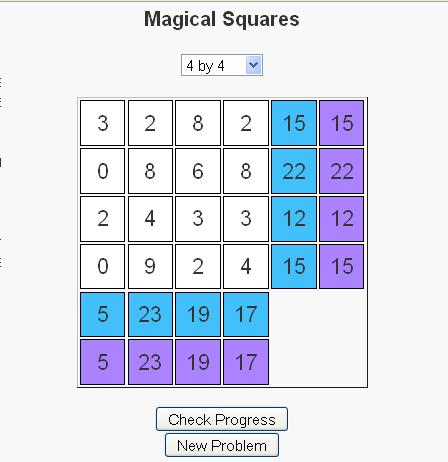# Triangle Sum Puzzle

This is probably a consequence of being a mathematician, but I have always enjoyed number puzzles. I think that there is a general simplicity and universality in numbers that are not present in things like word puzzles, where the ability to reach a solution can be limited to the vocabulary of the user.

The fact that these are puzzles and not simply homework exercises also helps because we often find people sharing difficulties and successes stories over the water cooler or at the lunch table. The fact that many of these math puzzles can teach some of the same concepts as homework problems (in a more fun and inclusive way) is generally lost on the user as their primary interest is generally on solving the puzzle in front of them, or sometimes solving the more general form of the puzzle.

Today’s post is about a puzzle that was originally shared with me over a lunch table by a friend who thought it was an interesting problem and asked what I thought about it. I didn’t give the puzzle much further thought (he had correctly solved the puzzle) until I saw it again in “Algorithmic Puzzles” by Anany Levitin and Maria Levitin. It was then that I thought about the more general form of the puzzle, derived a solution for the problem, and decided to code it up as a script for my site.

Below is a link to the puzzle:We have a set of random numbers arranged in a triangle and the question is to find the path of maximum sum from the root node (the top node) to the base (one of the nodes on the bottom row) with the rules that
(1) Exactly one number must be selected from each row
(2) A number can only be selected from a row if (a) it is the root node or (b) one of the two nodes above it has been selected.

For the sample
So for the sample problem in the picture, the maximal path would go through nodes 57, 99, 34, 95, and 27.

For more of these puzzles check out the script I write here and be sure to let me know what you think.

# Magical Squares Game

Whether introduced as children in elementary school, as adults in the workplace, or somewhere in between, the concept of magic squares has fascinated people for centuries; The Wikipedia article has discoveries of magic squares dating back to 650 B.C. in China.

Magic Squares of size n (for n >= 3) are n by n grids where the numbers 1, 2, …, n2 are specially arranged such that the sum of each row, column, and diagonal all sum to the same number. Below are two examples of magic squares of size 3 and 4.

 8 3 4 1 5 9 6 2 7
 4 1 16 13 15 11 2 6 10 14 7 3 5 8 9 12

Notice first, that in the first square all the numbers 1, 2, 3, .., 9 are used in the square. Likewise, the numbers 1, 2, …, 15, 16 are used in the second square. Second notice that each row, column and diagonal sums to 15 in the first square and 34 in the second square.

I have recently published a puzzle that is based on the concept of magic squares. There are some slight differences though.
– First, instead of using the numbers 1, 2, …, n2 a random set of numbers are generated.
– Second, the rows and columns each have a desired sum that we would like the numbers in the row/column to sum to.These two changes allow for a puzzle concept to be formulated based on the magic square concept. Users take turns swapping elements until the numbers in each row and column sum to the number in their goal cell, which is located in the last column or row of the grid. Above is an image of a solved puzzle.

Users can determine their progress the numbers in the next-to-last column, which tells the current sum of the numbers in that respective row or column.

To swap two numbers, first click on a cell with one of the two numbers in it. The cell should then turn red. Then click on the cell with the other number in it and the numbers should swap. If you click on the same cell twice no action should take place (except for the cell to turn red and then blank again).

Take a moment to check out the puzzles and let me know what you think.

# Arithmetic SequencesAt a previous job of mine, there was a policy of holding a dinner party for the company each time we hired a new employee. At these dinners, each employee was treated to a \$20 dinner at the expense of the company. There was also a manager responsible for keeping track of the costs of these dinners.

In computing the costs, the manager noticed that each time there is a new dinner, it was \$20 more expensive than the last one. So if we let a1 represent the cost of the first dinner, and let ai represent the cost of the ith dinner, then we see that ai = ai-1 + 20. Sequences like this, where t arise quite often in practice and are called arithmetic sequences. An arithmetic sequence is a list of numbers where the difference between any two consecutive numbers is constant.

For the example above, the term an will represent the cost of dinner after the nth employee has joined the company (assuming that no employees have left the company over this time period). Also the term Sn will represent the total cost the company has paid towards these dinners.

Before we continue with this example, consider the following table which lists the first five terms of an arithmetic sequence as well as the common difference and the first five sums of this sequence.

 term number term value diff sum number sum value a1 4 3 S1 4 a2 7 3 S2 11 a3 10 3 S3 21 a4 13 3 S4 34 a5 16 3 S5 50

One of the beauties of arithmetic sequences is that if we know the first term (a1) and the common difference (d), then we can easily calculate the terms an and Sn for any n with the following formulas:

an = a1 + d*(n – 1), where d is the common difference.
Sn = n*(a1 + an)/2

We can use these formulas to derive more information about the sequence. For example, if my manager wanted to estimate the cost of dinners once we had added 30 new employees, this would be term a30 of the sequence, which we can evaluate with the above formula by a30 = a1 + d*(n – 1) = 0 + 20*(30 – 1) = 0 + 20 * 29 = 580.

The script is available at http://www.learninglover.com/examples.php?id=33.

Other Blogs that have covered this topic:
Study Math Online

# Fraction Arithmetic

Fraction Arithmetic

I hope everyone had a good holiday season. I certainly enjoyed mine. Over this season, I had a chance to speak with some youth and their parents. Funny that whenever we discuss that I have a PhD in applied mathematics, the topics of the children struggling in mathematics and the possibility of tutoring their children always seem to come up. I have no problem with tutoring and I actively participate in such sessions in my spare time. However I will say that it is sometimes a difficult task to do this job over such a short time period. Needless to say, I felt bad that I couldn’t have been of more assistance.

So, this being the holiday season and all, I decided to make somewhat of a new years resolution to focus this site more towards some of the things that the youth struggle with to hopefully be able to answer some of their questions.

With that being said, the first area that I decided to look at was fractions. This is one of the first areas where the youth begin to dislike mathematics. I feel like regardless of how much teachers and professors speak of the importance of understanding these processes, many students simply never grasp the procedures involved, partially because they never get used to the rules associated with these matters.

In this first script on fractions, I’ve focused on four types of problems corresponding to the four basic operations of arithmetic: Addition, Subtraction, Multiplication and Division.

To add two fractions of the form

 num1 den1
+
 num2 den2

We use the formula

 num1 den1
+
 num2 den2
=
 num1 den1
+
 num2 den2
=
 num1*den2 + num2*den1 den1*den2

Lets take a moment to consider where this formula comes from. In order to be able to add fractions we first need to obtain a common denominator for the two fractions. One way that always works to obtain a common denominator is to multiply the denominators of the two fractions. So in the formula above, the denominator on the right hand side of the equals sign is the product of the two denominators on the left hand side. Once we have a common denominator, we need to rewrite each of the two fractions in terms of this common denominator.

 num1 den1
+
 num2 den2
=
 num1*den2 den1*den2
+
 num2*den1 den1*den2

The formula for subtracting fractions is similar, with the notable difference of a subtraction in the place of addition.

 num1 den1
 num2 den2
=
 num1*den2 – num2*den1 den1*den2

To multiply two fractions (also known as taking the product of two fractions, the resulting numerator is the product of the two initial numerators, and likewise the resulting denominator is the product of the two initial denominators.

 num1 den1
*
 num2 den2
=
 num1*num2 den1*den2

Finally, remembering that division is the inverse of multiplication, we can derive the formula to divide two fractions by multiplying by the inverse of the fractions:

 num1 den1
÷
 num2 den2
=
 num1 den1
*
 den2 num2
=
 num1*den2 den1*num2

The next step in each of these operations is to reduce the fraction to lowest terms. One way of doing this is by considering Euclid’s GCD algorithm which is available here.

The script is available to practice your work on fractions at
http://www.learninglover.com/examples.php?id=31